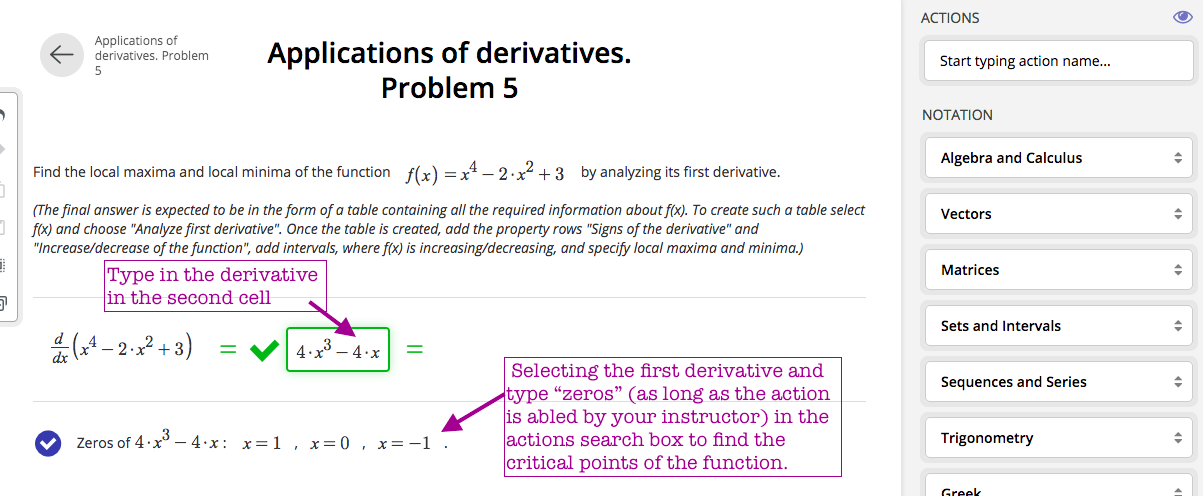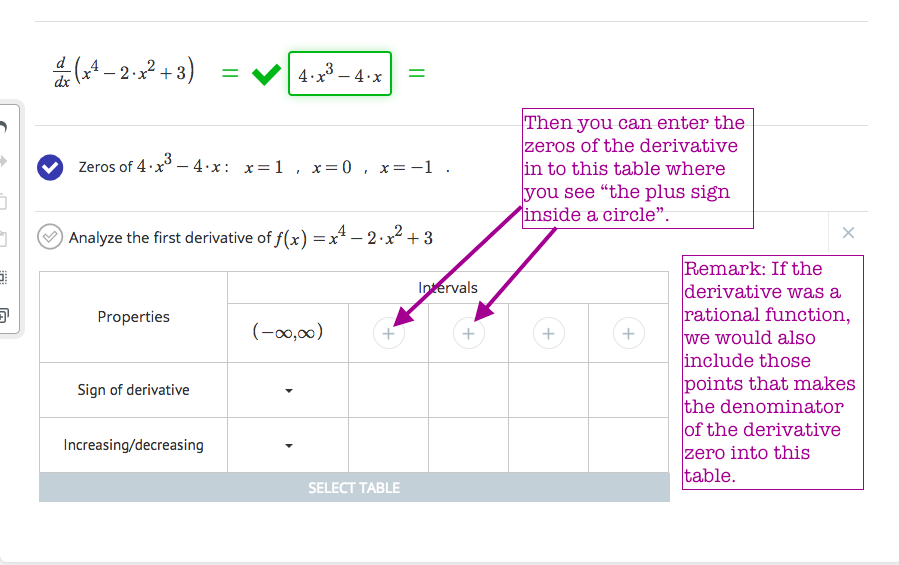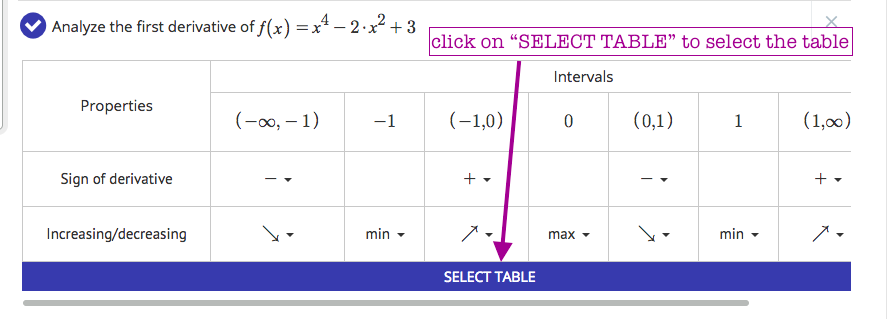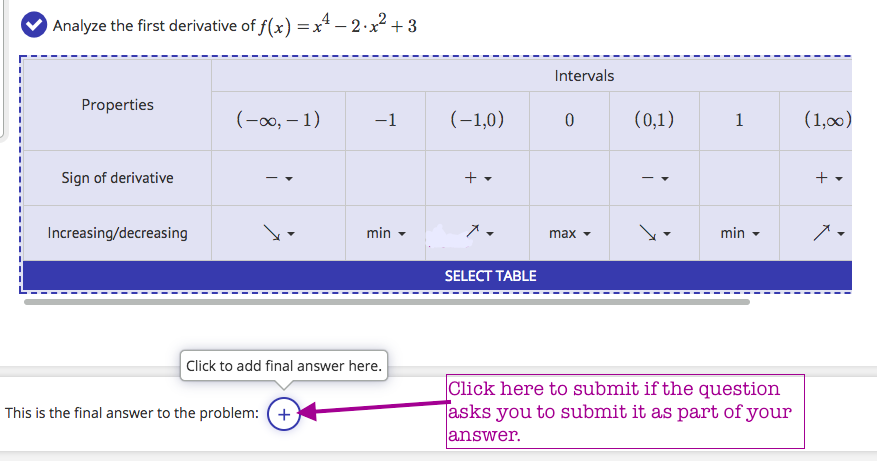"Analyze first derivative" action is very useful for finding interval of increase and decrease of a function as well as finding its local maxima and minima. In other words, it is a simple and organized way of analyzing the sign of the first derivative of a function.

Note that the steps followed below might be slightly different if the given function is not a polynomial. Here we described the basic idea on how to use the "Analyze first derivative action".  If you need further help with this action, please do not hesitate to contact us here.

• First find the derivative of the function:• Compute the critical points. (The points in the domain of the function where the first derivative is either zero or not defined):• Find the action "Analyze first derivative" after selecting the function:• Enter the points where derivative might be switching signs:• Decide the sign of the first derivative, then decide the intervals where the function is increasing and decreasing according to the sign of the derivative (negative derivative means function is decreasing, positive derivative means function is increasing). Finally decide the local maxima and minima of the function using the first derivative test.